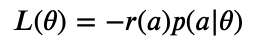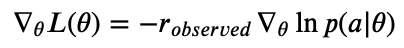1595968800

# Simultaneous Continuous/Discrete Hyperparameter Tuning

## Overview

In my previous article, I showed how to build policy gradients from scratch in Python, and we used it to tune discrete hyperparameters for machine learning models. (If you haven’t read it already, I’d recommend starting there.) Now, we’ll build on that progress, and extend policy gradients to optimize continuous parameters as well. By the end of this article, we’ll have a full-fledged method for simultaneously tuning discrete and continuous hyperparameters.

From last time, recall that policy gradients optimizes the following cost function for tuning hyperparameters:where a is the set of hyperparameters chosen for a particular experiment, and theta represents all trainable parameters for our PG model. Then, p denotes the probability of selecting action a, and r is the “reward” received for that action. We then showed that:The above equation tells us how to update our PG model, given a set of actions and their observed _rewards. _For discrete hyperparameters, we directly updated the relative log-probabilities (logits) for each possible action:

``````from typing import Sequence, Dict, Callable

import numpy as np
from numpy import ndarray

def softmax(x: ndarray, axis: int = -1) -> ndarray:
"""Computes the probability for selecting each discrete value."""
return np.exp(x) / np.sum(np.exp(x), axis=axis, keepdims=True)

class CategoricalActor:
def __init__(self, dim: int):
self.dim = dim
# Relative log-probabilities for selecting each discrete value.
# Initialize to equal weights of 'log(1 / dim)'.
self.logits = -np.log(dim) * np.ones(dim)

def action(self) -> ndarray:
"""Performs a weighted draw of the discrete values, using 'self.logits'."""
return np.argmax(softmax(self.logits) * np.random.rand(self.dim))

def update(self, actions: ndarray, values: ndarray, lr: float = 0.1) -> None:
"""Given a batch of actions (hyperparameters) and their relative values, update
the model's internal parameters (logits) to maximize future action values."""
# Normalize values, so scaling the cost function doesn't affect training.
values = (values - values.mean()) / (values.std() + 1e-5)
values = values.reshape(-1, 1)

# Mask gradients, so that we only update parameters that were selected in 'actions'
mask = np.arange(len(self.logits)).reshape(1, -1) == actions.reshape(-1, 1)
# Gradient of log-softmax is (1 - softmax).  Go ahead and multiply by 'values'.
grads = -values * (1 - softmax(self.logits)).reshape(1, -1)
# Compute gradient for each logit.  Don't average over entries that were masked
# out, and avoid dividing by zero.

view raw
categorical_actor.py hosted with ❤ by GitHub
``````

This approach will not work for continuous hyperparameters, because we cannot possibly store the log-probability for every possible outcome! We need a new method for generating continuous random variables and their relative log-probabilities.

## Extending to Continuous Hyperparameters

In the field of reinforcement learning, continuous variables are commonly modeled using Gaussian Processes. The idea is pretty straightforward: our model predicts the mean and standard deviation for a Gaussian distribution, and we gather actions/predictions using a random number generator.

#optimization #ai #reinforcement-learning #machine-learning #neural-networks #deep learning

## Buddha Community1597275960

## Hyperparameters Tuning Using GridSearchCV And RandomizedSearchCV

While building a Machine learning model we always define two things that are model parameters and model hyperparameters of a predictive algorithm. Model parameters are the ones that are an internal part of the model and their value is computed automatically by the model referring to the data like support vectors in a support vector machine. But hyperparameters are the ones that can be manipulated by the programmer to improve the performance of the model like the learning rate of a deep learning model. They are the one that commands over the algorithm and are initialized in the form of a tuple.

In this article, we will explore hyperparameter tuning. We will see what are the different parts of a hyperparameter, how it is done using two different approaches – GridSearchCV and RandomizedSearchCV. For this experiment, we will use the Boston Housing Dataset that can be downloaded from Kaggle. We will first build the model using default parameters, then we will build the same model using a hyperparameter tuning approach and then will compare the performance of the model.

1. What is Hyper Parameter Tuning?
2. What steps to follow to do Hyper Parameter Tuning?
3. Implementation of Regression Model
4. Implementation of Model using GridSearchCV
5. Implementation of Model using RandomizedSearchCV
6. Comparison of Different Models

#### 1. What Is Hyperparameter Tuning?

Hyperparameter tuning is the process of tuning the parameters present as the tuples while we build machine learning models. These parameters are defined by us which can be manipulated according to programmer wish. Machine learning algorithms never learn these parameters. These are tuned so that we could get good performance by the model. Hyperparameter tuning aims to find such parameters where the performance of the model is highest or where the model performance is best and the error rate is least. We define the hyperparameter as shown below for the random forest classifier model. These parameters are tuned randomly and results are checked.

#developers corner #hyperparameter tuning #hyperparameters #machine learning #parameter tuning1600966800

## Hyperparameter tuning with Keras and Ray Tune

In my previous article, I had explained how to build a small and nimble image classifier and what are the advantages of having variable input dimensions in a convolutional neural network. However, after going through the model building code and training routine, one can ask questions such as:

1. How to choose the number of layers in a neural network?
2. How to choose the optimal number of units/filters in each layer?
3. What would be the best data augmentation strategy for my dataset?
4. What batch size and learning rate would be appropriate?

Building or training a neural network involves figuring out the answers to the above questions. You may have an intuition for CNNs, for example, as we go deeper the number of filters in each layer should increase as the neural network learns to extract more and more complex features built on simpler features extracted in the earlier layers. However, there might be a more optimal model (for your dataset) with a lesser number of parameters that might outperform the model that you have designed based on your intuition.

In this article, I’ll explain what these parameters are and how do they affect the training of a machine learning model. I’ll explain how do machine learning engineers choose these parameters and how can we automate this process using a simple mathematical concept. I’ll be starting with the same model architecture from my previous article and will be modifying it to make most of the training and architectural parameters tunable.

#data-science #machine-learning #deep-learning #hyperparameter-tuning #bayesian-optimization1598062920

## Fine Tuning XGBoost model

Tuning the model is the way to supercharge the model to increase their performance. Let us look into an example where there is a comparison between the untuned XGBoost model and tuned XGBoost model based on their RMSE score. Later, you will know about the description of the hyperparameters in XGBoost.

Below is the code example for untuned parameters in XGBoost model:

``````#Importing necessary libraries
import pandas as pd
import numpy as np
import xgboost as xg

X,y = house[house.columns.tolist()[:-1]],
house[house.columns.tolist()[-1]]

#Converting it into DMatrix
house_dmatrix = xgb.DMatrix(data = X, label = y)

#Parameter configuration
param_untuned = {"objective":"reg:linear"}

cv_untuned_rmse = xg.cv(dtrain = house_dmatrix, params = param_untuned, nfold = 4,
metrics = "rmse", as_pandas = True, seed= 123)
print("RMSE Untuned: %f" %((cv_untuned_rmse["test-rmse-mean"]).tail(1)))
view raw
tune_1.py hosted with ❤ by GitHub
``````

Output: 34624.229980

Now let us look to the value of RMSE when the parameters are tuned to some extent:

``````#Importing necessary libraries
import pandas as pd
import numpy as np
import xgboost as xg

X,y = house[house.columns.tolist()[:-1]],
house[house.columns.tolist()[-1]]

#Converting it into DMatrix
house_dmatrix = xgb.DMatrix(data = X, label = y)

#Parameter Configuration
param_tuned = {"objective":"reg:linear", 'colsample_bytree': 0.3,
'learning_rate': 0.1, 'max_depth': 5}

cv_tuned_rmse = xg.cv(dtrain = house_dmatrix, params = param_tuned, nfold = 4,
num_boost_round = 200, metrics = "rmse", as_pandas = True, seed= 123)
print("RMSE Tuned: %f" %((cv_tuned_rmse["test-rmse-mean"]).tail(1)))
view raw
tune_2.py hosted with ❤ by GitHub
``````

Output: 29812.683594

It can be seen that there is around 15% reduction in the RMSE score when the parameters got tuned.

#machine-learning #hyperparameter #artificial-intelligence #hyperparameter-tuning #xgboost #deep learning1600891200

## A Novice’s Guide to Hyperparameter Optimization at Scale

Despite the tremendous success of machine learning (ML), modern algorithms still depend on a variety of free non-trainable hyperparameters. Ultimately, our ability to select quality hyperparameters governs the performance for a given model. In the past, and even some currently, hyperparameters were hand selected through trial and error. An entire field has been dedicated to improving this selection process; it is referred to as hyperparameter optimization (HPO). Inherently, HPO requires testing many different hyperparameter configurations and as a result can benefit tremendously from massively parallel resources like the Perlmutter system we are building at the National Energy Research Scientific Computing Center (NERSC). As we prepare for Perlmutter, we wanted to explore the multitude of HPO frameworks and strategies that exist on a model of interest. This article is a product of that exploration and is intended to provide an introduction to HPO methods and guidance on running HPO at scale, based on my recent experiences and results.

Disclaimer; this article contains plenty of general non-software specific information about HPO, but there is a bias for free open source software that is applicable to our systems at NERSC.

#editors-pick #machine-learning #hyperparameter #hyperparameter-tuning #deep-learning1595968800

## Overview

In my previous article, I showed how to build policy gradients from scratch in Python, and we used it to tune discrete hyperparameters for machine learning models. (If you haven’t read it already, I’d recommend starting there.) Now, we’ll build on that progress, and extend policy gradients to optimize continuous parameters as well. By the end of this article, we’ll have a full-fledged method for simultaneously tuning discrete and continuous hyperparameters.

From last time, recall that policy gradients optimizes the following cost function for tuning hyperparameters:where a is the set of hyperparameters chosen for a particular experiment, and theta represents all trainable parameters for our PG model. Then, p denotes the probability of selecting action a, and r is the “reward” received for that action. We then showed that:The above equation tells us how to update our PG model, given a set of actions and their observed _rewards. _For discrete hyperparameters, we directly updated the relative log-probabilities (logits) for each possible action:

``````from typing import Sequence, Dict, Callable

import numpy as np
from numpy import ndarray

def softmax(x: ndarray, axis: int = -1) -> ndarray:
"""Computes the probability for selecting each discrete value."""
return np.exp(x) / np.sum(np.exp(x), axis=axis, keepdims=True)

class CategoricalActor:
def __init__(self, dim: int):
self.dim = dim
# Relative log-probabilities for selecting each discrete value.
# Initialize to equal weights of 'log(1 / dim)'.
self.logits = -np.log(dim) * np.ones(dim)

def action(self) -> ndarray:
"""Performs a weighted draw of the discrete values, using 'self.logits'."""
return np.argmax(softmax(self.logits) * np.random.rand(self.dim))

def update(self, actions: ndarray, values: ndarray, lr: float = 0.1) -> None:
"""Given a batch of actions (hyperparameters) and their relative values, update
the model's internal parameters (logits) to maximize future action values."""
# Normalize values, so scaling the cost function doesn't affect training.
values = (values - values.mean()) / (values.std() + 1e-5)
values = values.reshape(-1, 1)

# Mask gradients, so that we only update parameters that were selected in 'actions'
mask = np.arange(len(self.logits)).reshape(1, -1) == actions.reshape(-1, 1)
# Gradient of log-softmax is (1 - softmax).  Go ahead and multiply by 'values'.
grads = -values * (1 - softmax(self.logits)).reshape(1, -1)
# Compute gradient for each logit.  Don't average over entries that were masked
# out, and avoid dividing by zero.